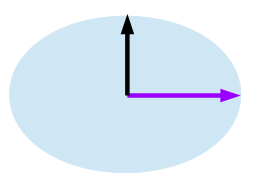# Ellipse Area CalculationAn ellipse is a curve that surrounds two points called focal points. For any point on the curve, the sum of the distance from these two points is constant.

An ellipse has two radii (we name α and β). The area of the ellipse is calculated in the following;

π x α x β

Ellipse Area:

Updated:

Tags:   math